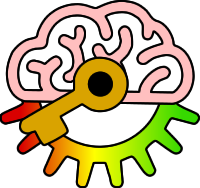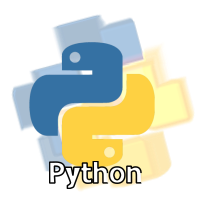# Y8

6g: Use relational operators to form logical expressions

Keywords:Test your self on these keywords and definitions using the games below or
KeywordDefinition
!=relational operator which means "not equal to"
<relational operator which means "less than"
<=relational operator which means "less than or equal to"
==relational operator which means "equal to"
>relational operator which means "greater than"
>=relational operator which means "greater than or equal to"
logical expressiona value which can only be one of either true or false
relational operatorsymbol or symbols which are used to compare two values
Keyword games:
Resources: